# How to☝️ Divide in Google Sheets

To divide in Google Sheets, select a cell, go to the Formula bar, enter the formula =A1/B1, and hit Enter.

Scroll down to learn different methods on how you can divide values in just a few seconds. In this article, you will learn all the details related to division in Google Sheets.

## How to Divide in Google Sheets: Two Methods

Decide which method suits you best by checking out both. Let’s get down to business!

### Method 1: Using the Division Operator

Start out by trying the easiest method to divide, described below. Let’s take a look!

Difficulty: Beginner

Time Estimate: 5 seconds

1. Click on a blank cell.
2. Go to the Formula bar and type the formula =A1/B1, where A1 is the cell with the value to be divided (dividend), and B1 is the divisor.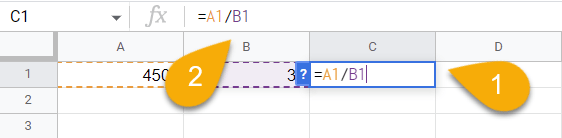3. Finally, press the Enter key on your keyboard.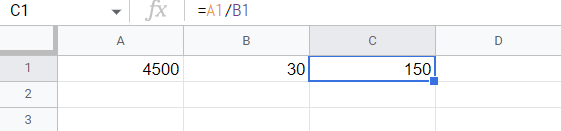Easy as ABC!

### Method 2: Using the DIVIDE Function

Let’s see the step-by-step guide for the second method:

Difficulty: Beginner

Time Estimate: 6 seconds

1. Select the blank cell where you need the result.
2. Navigate to the Formula bar and enter the formula =DIVIDE(A1, B1). Here A1 is the cell with the value to be divided (dividend), and B1 is the cell with the divisor.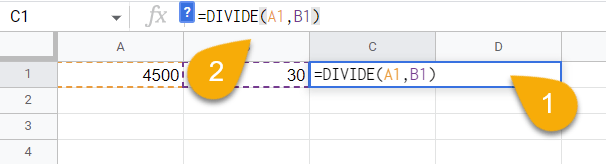3. Press Enter.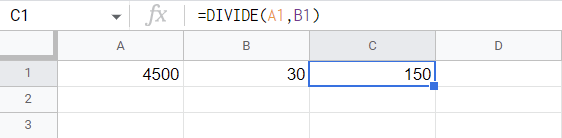That’s it!

In the next section, we will explore how this function works in detail.

#### Description

The DIVIDE function is a built-in function in Google Sheets that allows you to divide two numbers.

#### Purpose

This is useful when you want to evenly divide an amount (such as a sum of money) among a number of workers, determine income for a certain month or year, split a number of things among a group of people, and so on.

#### Syntax

=DIVIDE(dividend, divisor)

#### Arguments

• a dividend is the value to be divided.
• a divisor is the value to divide by.

#### Return Value

The result of division is an integer quotient. By dividing the first number by the second number, you get the number of times the second number is encapsulated within the first number.

#### Usage Notes

1. There can be no divisor equal to 0 in this equation. In the case of division by 0, an error appears in the cell (#DIV/0!).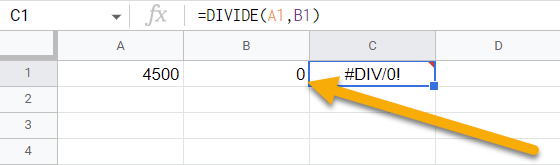2. Instead of the DIVIDE function, you can use the QUOTIENT function and the result will be the same.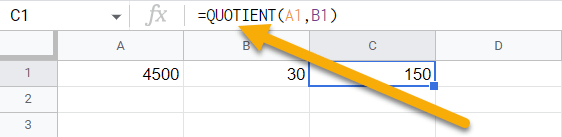3. It is possible to use numeric values instead of cell references in a formula.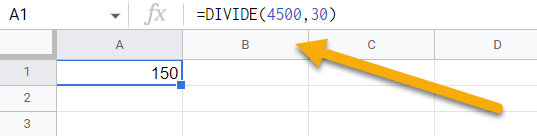4. Be sure to use the correct division sign. The backslash (\) will not execute your action. That’s why you need to use the forward-slash (/).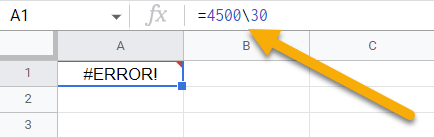#### Examples

The purpose of this section is to discuss examples of how this function can be used. So let’s get started!

1. Use the forward-slash (/) to divide the values in cells:

=A1/B1 // Output: 150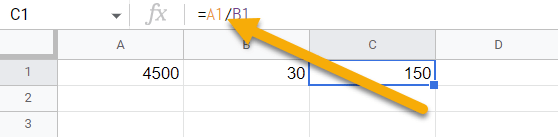1. Use the forward-slash (/) to divide numbers directly:

=4500/30 // Output: 150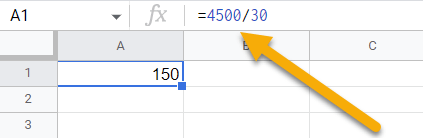1. Apply the DIVIDE function to divide the values in cells:

=DIVIDE(A1,B1) // Output: 150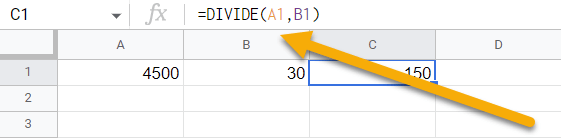1. Numbers can be divided using the DIVIDE function:

=DIVIDE(4500,30) // Output: 150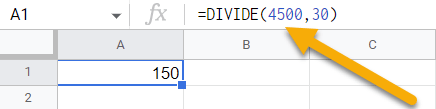1. To divide values in a cell, you can use the QUOTIENT function:

=QUOTIENT(A1,B1) // Output: 150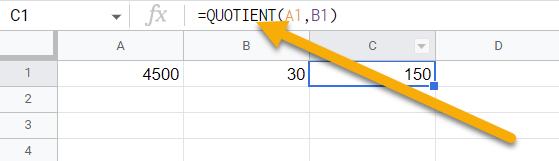1. For dividing two numbers, use the QUOTIENT function:

=QUOTIENT(4500,30) // Output: 150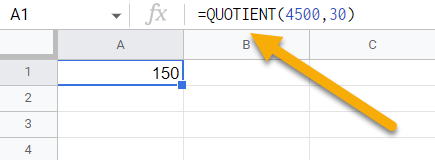This part of the article is highly recommended for those who would like to explore this topic in greater depth.

### How to Divide Multiple Cells

If you have to deal with multiple cells, then you might be interested in this method for division. Check it out!

1. Click on a blank cell.
2. Navigate to the Formula bar and enter the formula =A1/B1/C1. A1, B1, and C1 are cells with values.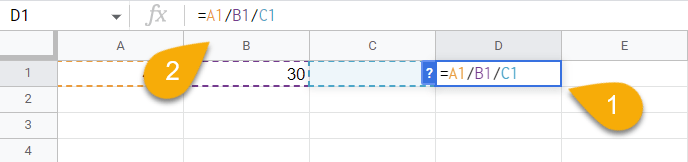3. Hit the Enter key.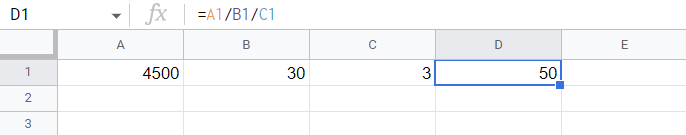Voila! The division process will happen from left to right: 4500/30=150/3=50.

### How to Divide Percentages

Find out how you can divide percentages easily by using the following tip:

1. Choose the cell where you want the result.
2. Type the formula =A1/B1 into the Formula bar.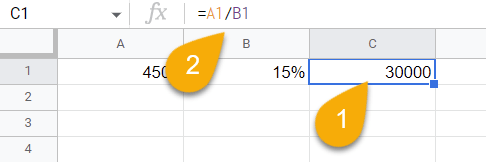3. Press Enter.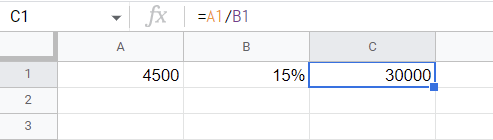Piece of cake!

### How to Divide Columns

If you have a large number of values in multiple columns that need to be divided between each other, use the following guide:

1. Select a blank cell.
2. Go to the Formula bar, and use the formula =A1/B1. A1 and B1 contain your values.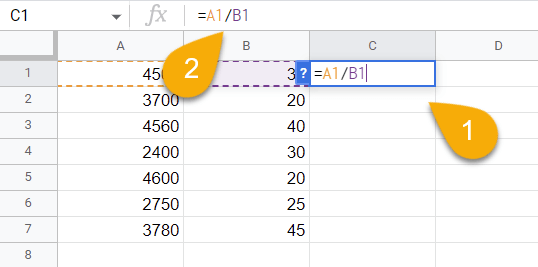3. Press the Enter key on your keyboard.
4. Lastly, drag the cell with the result downward to apply the division formula for the whole column.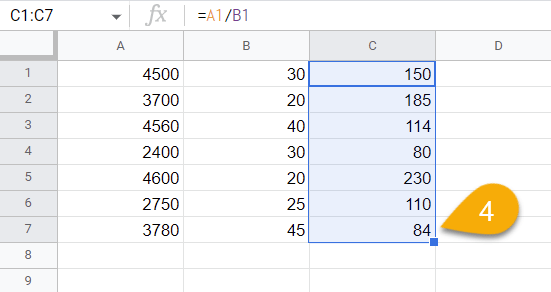Easy as ABC!

### How to Divide a Whole Column by One Number

If you need to divide all the values in a column by one number, you can do so by following the steps below:

1. Choose a blank cell where you want the result.
2. Navigate to the Formula bar and enter the formula =A1/\$B\$1, where A1 is the dividend, and \$B\$1 is the divisor.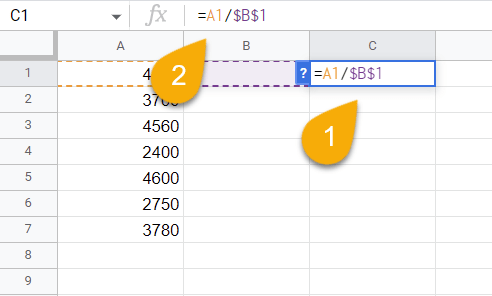3. Press Enter.
4. If you wish to divide the entire column, drag the cell with the result down.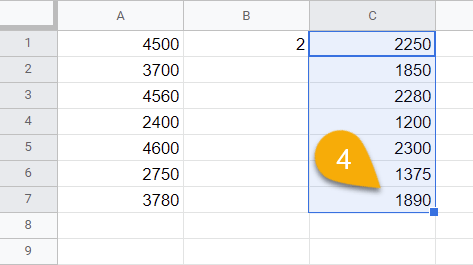And here is your result! The dollar signs keep the divisor (B1) the same even when the dividend (A1:A7) changes.

## How to Handle the #DIV/0! Error

The #DIV/0! error is generally caused when a formula attempts to divide by 0. This can happen when a cell containing a zero value is used as the divisor in a formula or a number format is applied to cells that contain 0 values.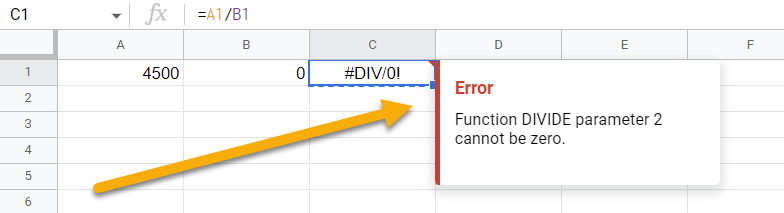If you need to correct the #DIV/0! Error, just check and fix the cell references with the 0 value. Change the values in your formula, and you will achieve the desired result.